# Lakhmir Singh Solutions For Class 8 Science Chapter 14 Chemical Effect Of Electric Current

The chemical changes which take place in conducting liquid on passing an electric current through them are called chemical effect on electric current. The material which allows electric current to pass through easily are called good conductors of electricity and those do not allow electric current is called poor conductors of electricity or non-conductors of electricity. For example when an electric current is passed through acidified water then bubbles of oxygen and hydrogen gases are produced at two electrodes. This is a chemical effect of electric current.

As solids conduct electricity, some of the liquids like acids, bases and salts also conduct electricity. When electricity is passed through a copper wire which is a solid, no chemical changes take place but when passed through an acidified water chemical changes take place in which water decomposes into hydrogen and oxygen gas.

## Download Lakhmir Singh Science Class 8 Solutions For Chapter 14 Chemical Effect Of Electric Current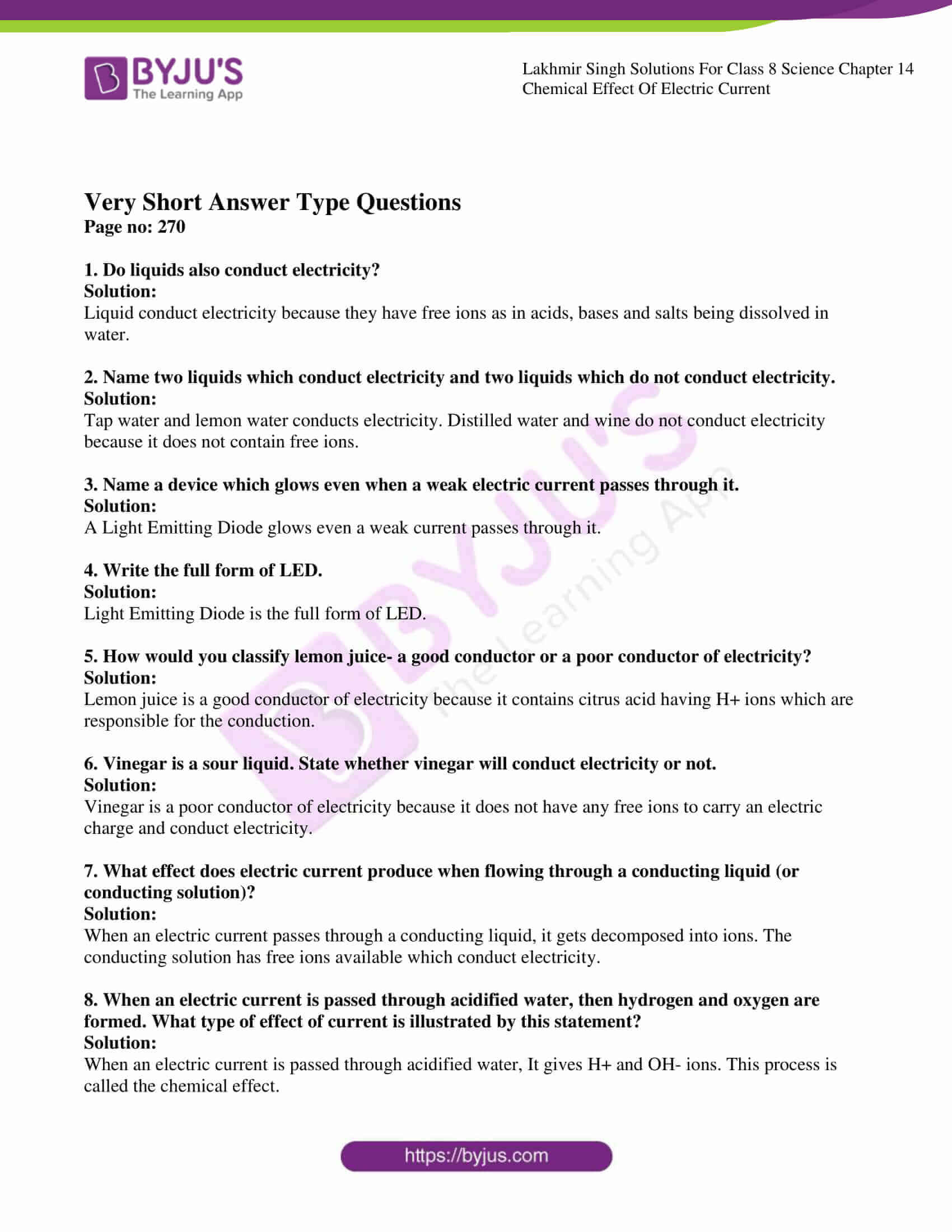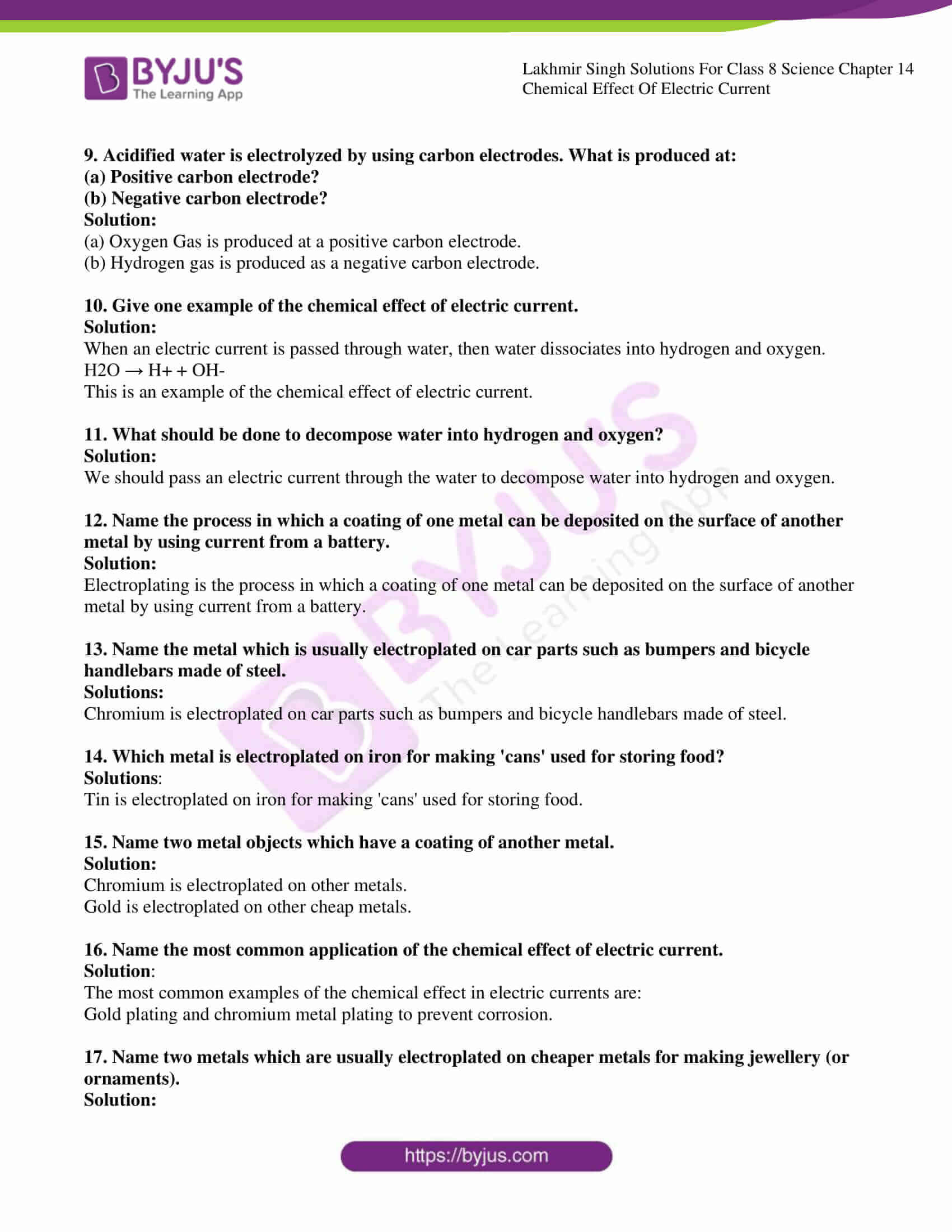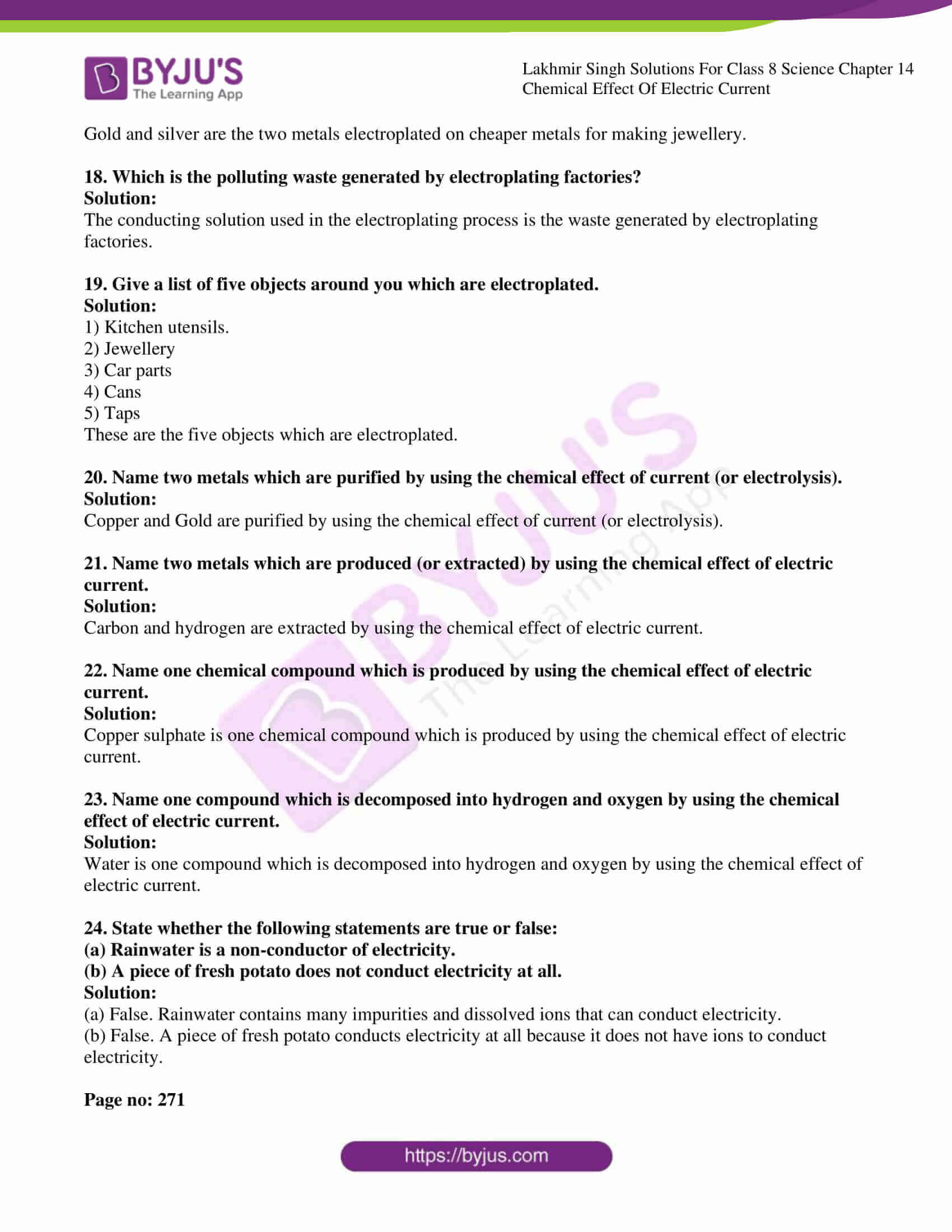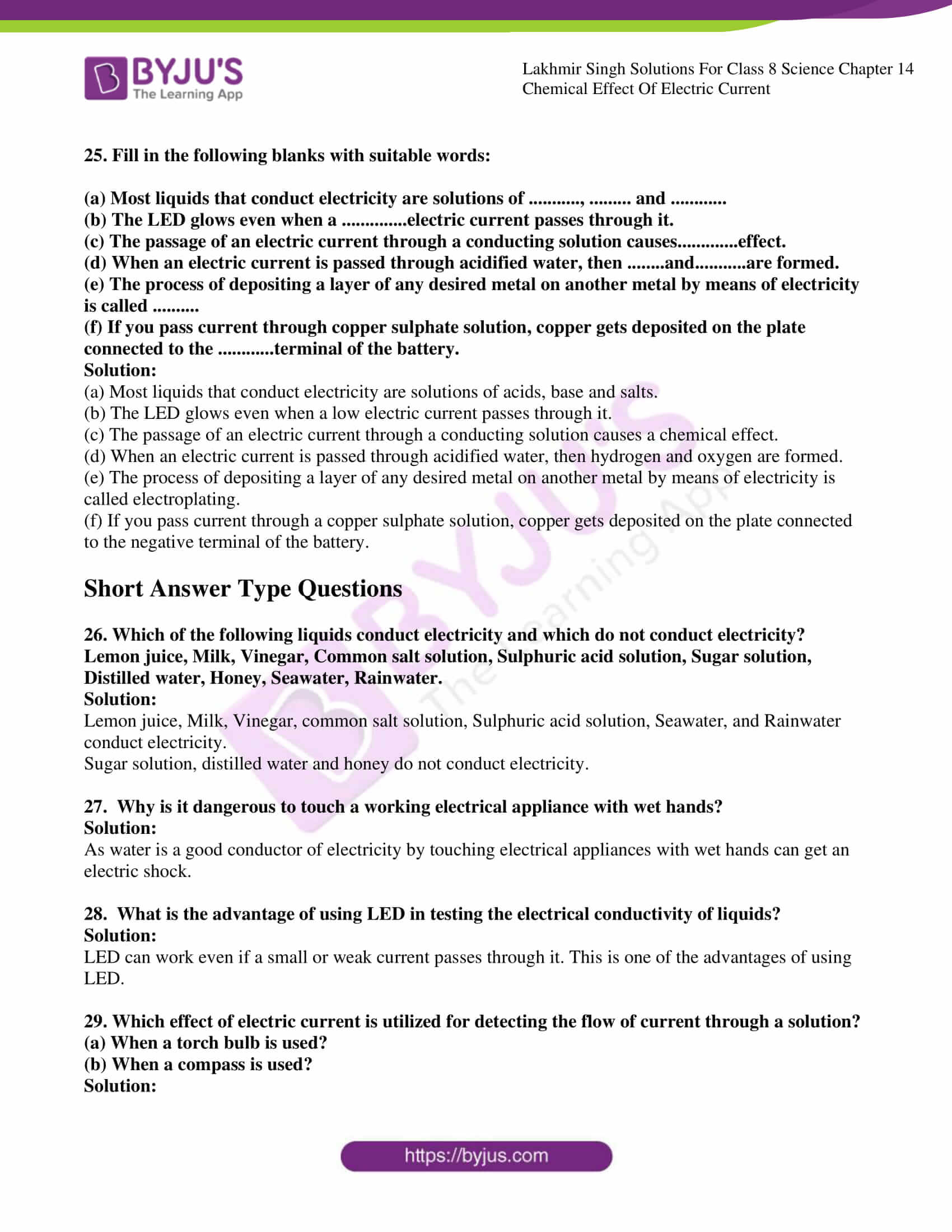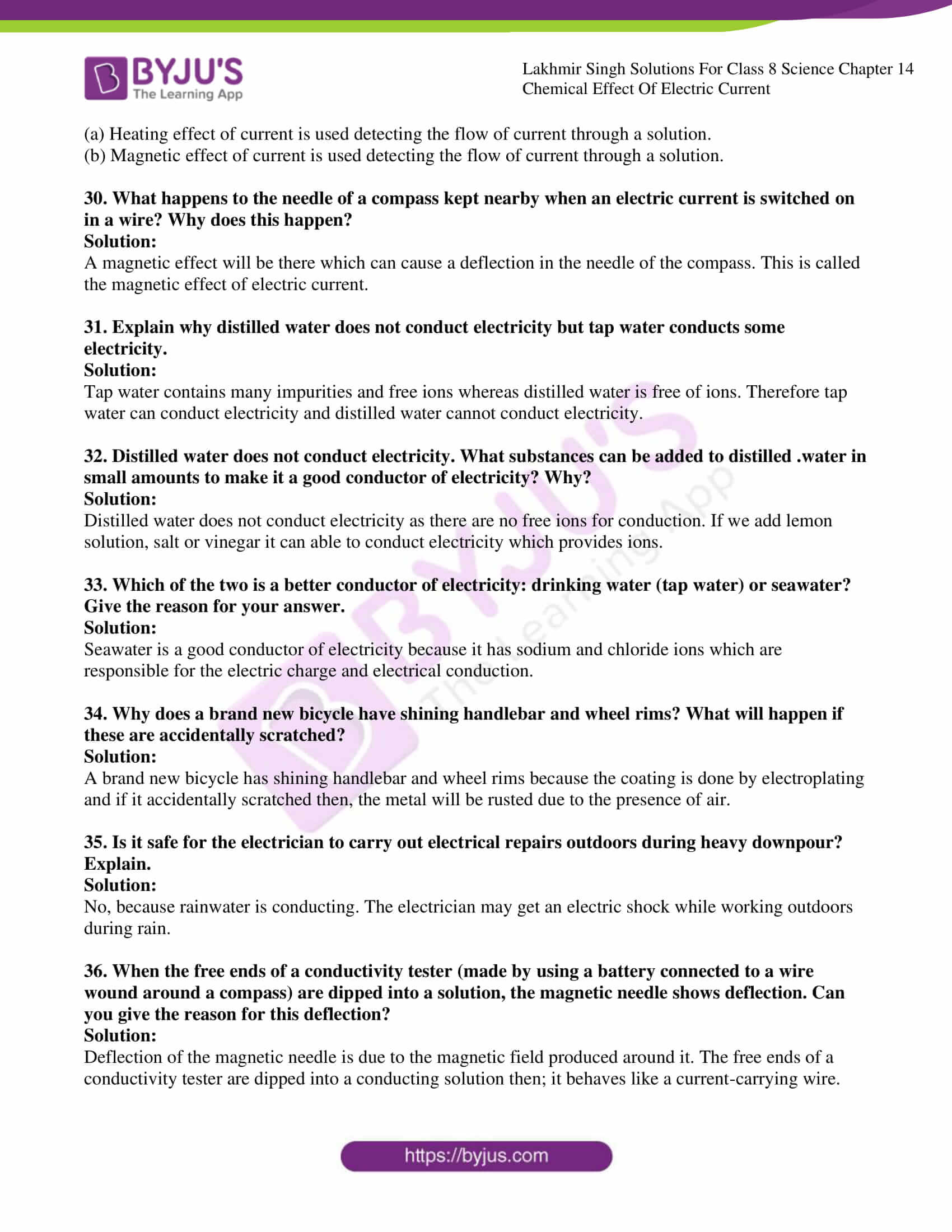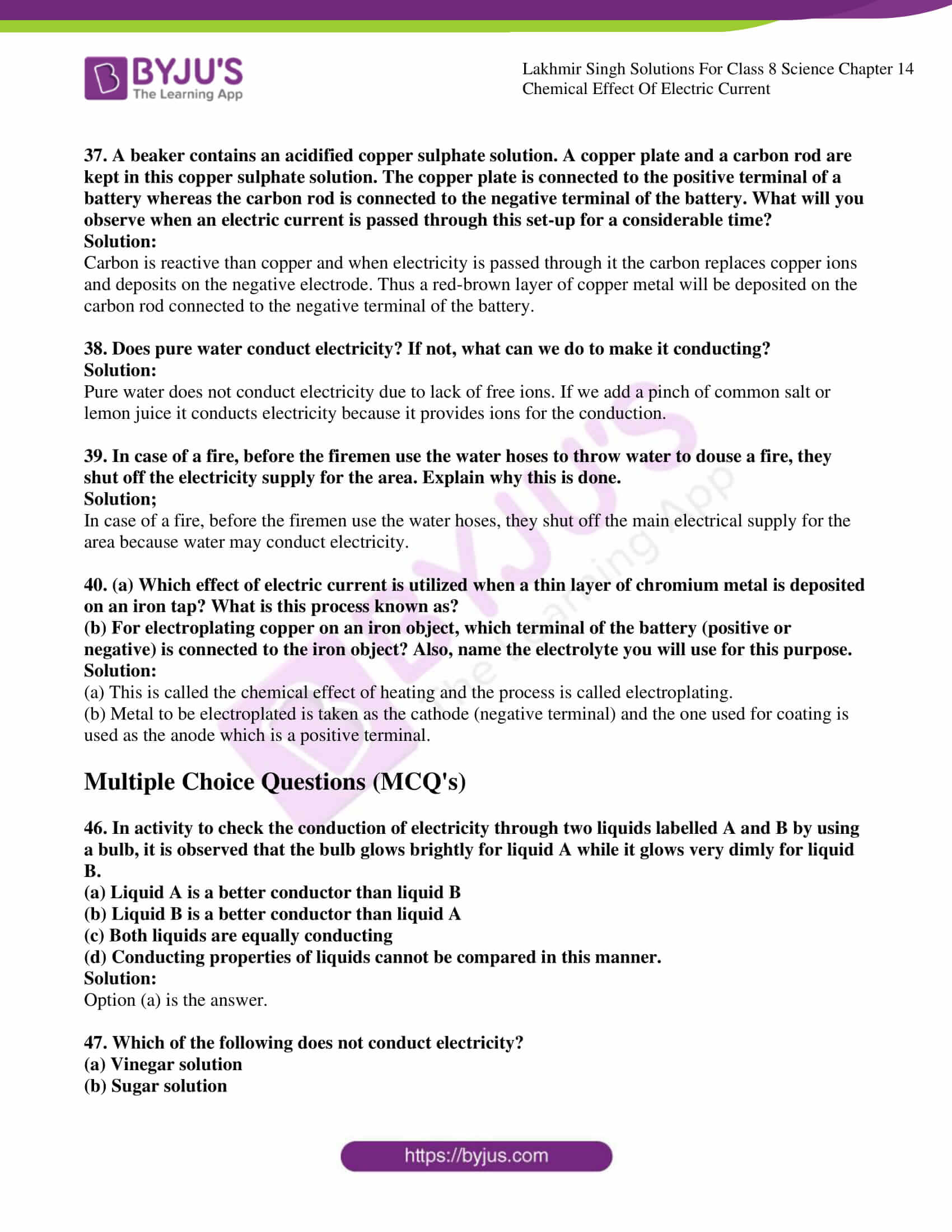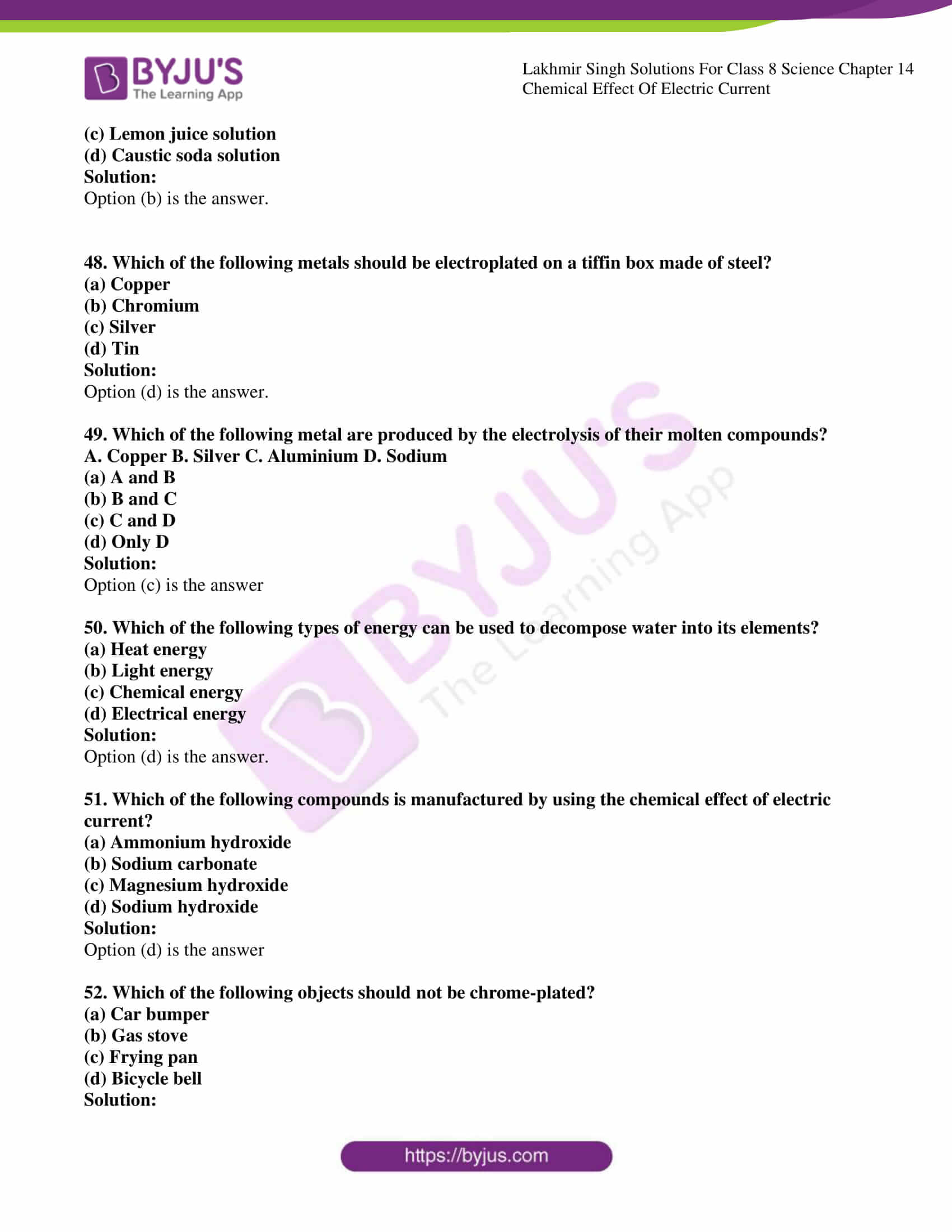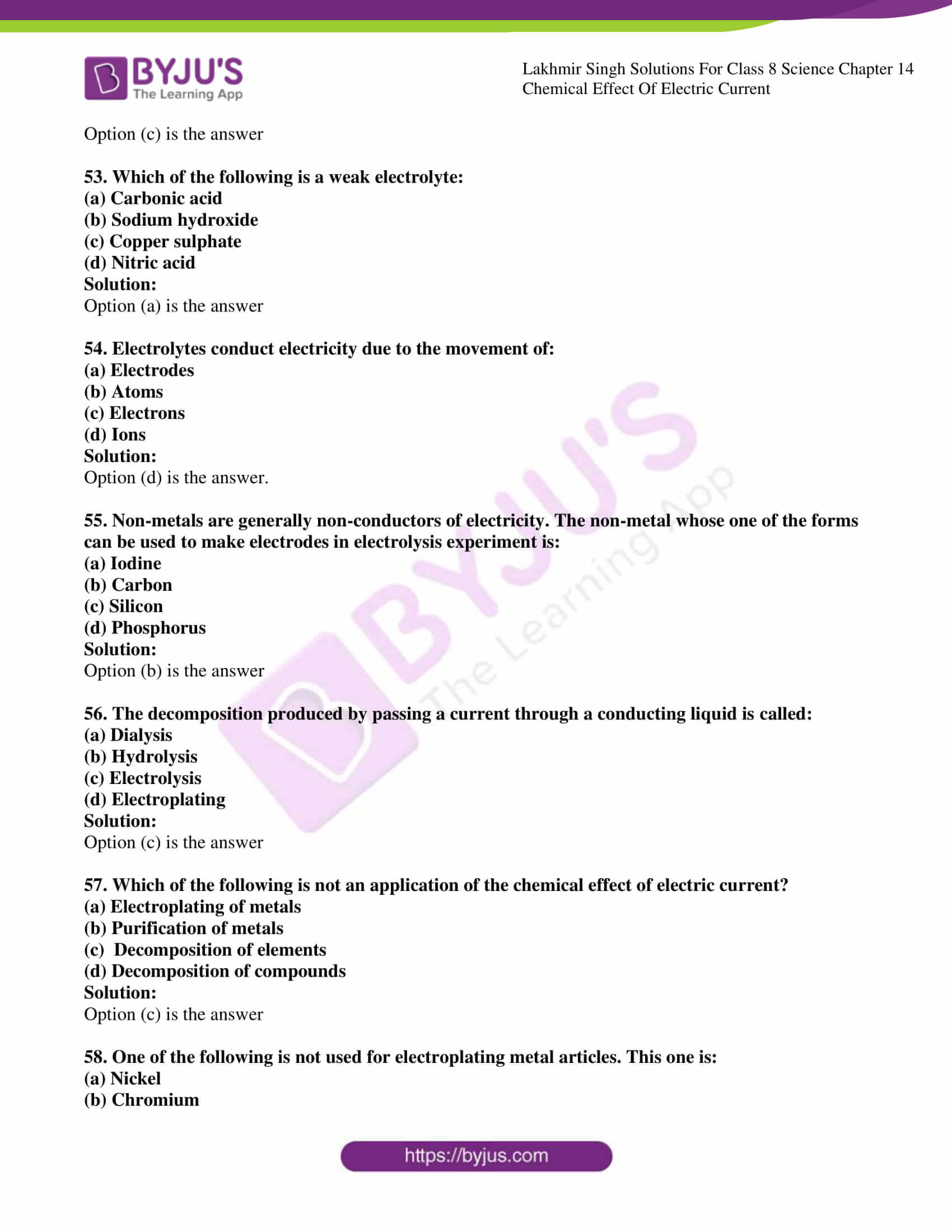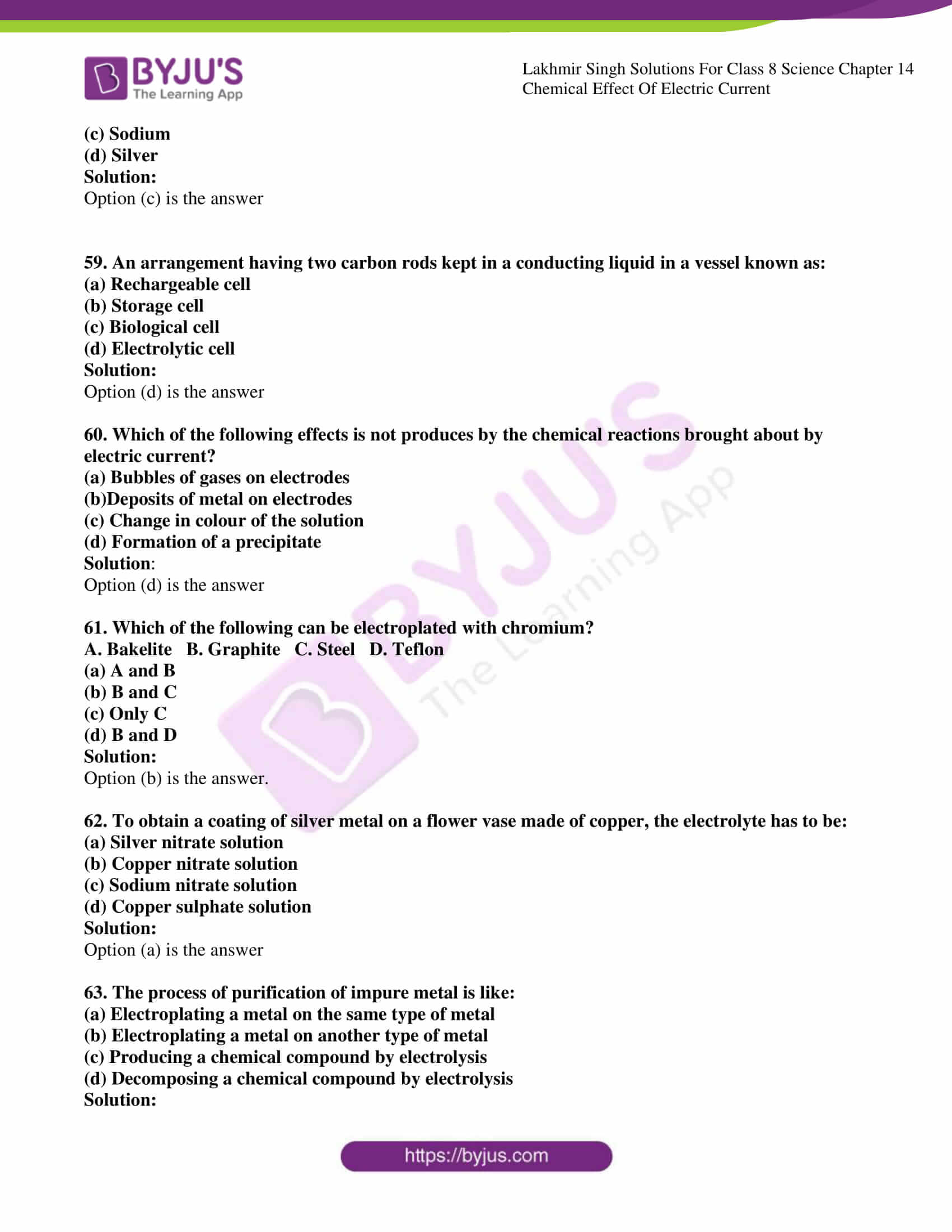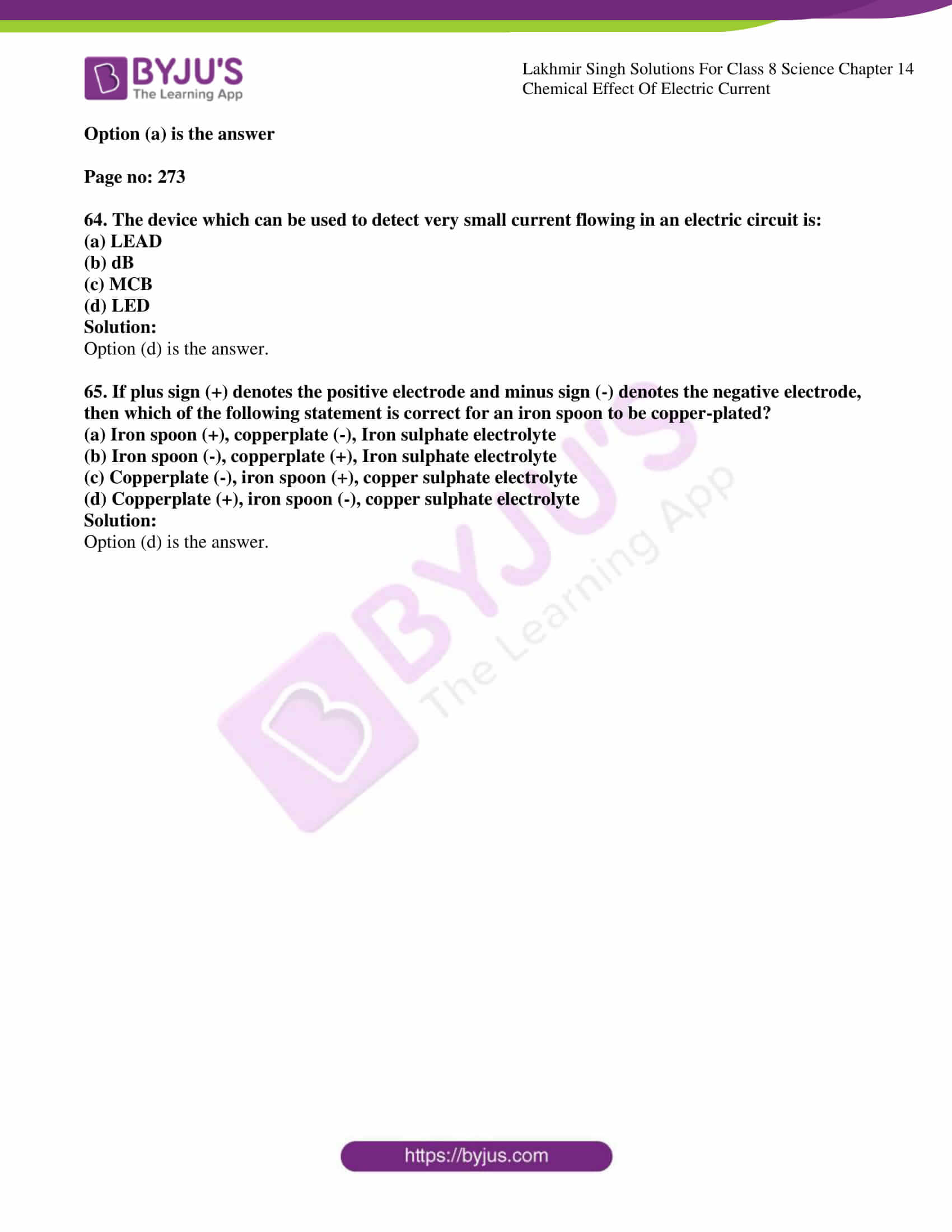### Access Lakhmir Singh Science Class 8 Solutions For Chapter 14

Page no: 270

1. Do liquids also conduct electricity?

Solution:

Liquid conduct electricity because they have free ions as in acids, bases and salts being dissolved in water.

2. Name two liquids which conduct electricity and two liquids which do not conduct electricity.

Solution:

Tap water and lemon water conducts electricity. Distilled water and wine do not conduct electricity because it does not contain free ions.

3. Name a device which glows even when a weak electric current passes through it.

Solution:

A Light Emitting Diode glows even a weak current passes through it.

4. Write the full form of LED.

Solution:

Light Emitting Diode is the full form of LED.

5. How would you classify lemon juice- a good conductor or a poor conductor of electricity?

Solution:

Lemon juice is a good conductor of electricity because it contains citrus acid having H+ ions which are responsible for the conduction.

6. Vinegar is a sour liquid. State whether vinegar will conduct electricity or not.

Solution:

Vinegar is a poor conductor of electricity because it does not have any free ions to carry an electric charge and conduct electricity.

7. What effect does electric current produce when flowing through a conducting liquid (or conducting solution)?

Solution:

When an electric current passes through a conducting liquid, it gets decomposed into ions. The conducting solution has free ions available which conduct electricity.

8. When an electric current is passed through acidified water, then hydrogen and oxygen are formed. What type of effect of current is illustrated by this statement?

Solution:

When an electric current is passed through acidified water, It gives H+ and OH- ions. This process is called the chemical effect.

9. Acidified water is electrolyzed by using carbon electrodes. What is produced at:

(a) Positive carbon electrode?

(b) Negative carbon electrode?

Solution:

(a) Oxygen Gas is produced at a positive carbon electrode.

(b) Hydrogen gas is produced as a negative carbon electrode.

10. Give one example of the chemical effect of electric current.

Solution:

When an electric current is passed through water, then water dissociates into hydrogen and oxygen.

H2O → H+ + OH-

This is an example of the chemical effect of electric current.

11. What should be done to decompose water into hydrogen and oxygen?

Solution:

We should pass an electric current through the water to decompose water into hydrogen and oxygen.

12. Name the process in which a coating of one metal can be deposited on the surface of another metal by using current from a battery.

Solution:

Electroplating is the process in which a coating of one metal can be deposited on the surface of another metal by using current from a battery.

13. Name the metal which is usually electroplated on car parts such as bumpers and bicycle handlebars made of steel.

Solutions:

Chromium is electroplated on car parts such as bumpers and bicycle handlebars made of steel.

14. Which metal is electroplated on iron for making ‘cans’ used for storing food?

Solutions:

Tin is electroplated on iron for making ‘cans’ used for storing food.

15. Name two metal objects which have a coating of another metal.

Solution:

Chromium is electroplated on other metals.

Gold is electroplated on other cheap metals.

16. Name the most common application of the chemical effect of electric current.

Solution:

The most common examples of the chemical effect in electric currents are:

Gold plating and chromium metal plating to prevent corrosion.

17. Name two metals which are usually electroplated on cheaper metals for making jewellery (or ornaments).

Solution:

Gold and silver are the two metals electroplated on cheaper metals for making jewellery.

18. Which is the polluting waste generated by electroplating factories?

Solution:

The conducting solution used in the electroplating process is the waste generated by electroplating factories.

19. Give a list of five objects around you which are electroplated.

Solution:

1) Kitchen utensils.

2) Jewellery

3) Car parts

4) Cans

5) Taps

These are the five objects which are electroplated.

20. Name two metals which are purified by using the chemical effect of current (or electrolysis).

Solution:

Copper and Gold are purified by using the chemical effect of current (or electrolysis).

21. Name two metals which are produced (or extracted) by using the chemical effect of electric current.

Solution:

Carbon and hydrogen are extracted by using the chemical effect of electric current.

22. Name one chemical compound which is produced by using the chemical effect of electric current.

Solution:

Copper sulphate is one chemical compound which is produced by using the chemical effect of electric current.

23. Name one compound which is decomposed into hydrogen and oxygen by using the chemical effect of electric current.

Solution:

Water is one compound which is decomposed into hydrogen and oxygen by using the chemical effect of electric current.

24. State whether the following statements are true or false:

(a) Rainwater is a non-conductor of electricity.

(b) A piece of fresh potato does not conduct electricity at all.

Solution:

(a) False. Rainwater contains many impurities and dissolved ions that can conduct electricity.

(b) False. A piece of fresh potato conducts electricity at all because it does not have ions to conduct electricity.

Page no: 271

25. Fill in the following blanks with suitable words:

(a) Most liquids that conduct electricity are solutions of ……….., ……… and …………

(b) The LED glows even when a …………..electric current passes through it.

(c) The passage of an electric current through a conducting solution causes………….effect.

(d) When an electric current is passed through acidified water, then ……..and………..are formed.

(e) The process of depositing a layer of any desired metal on another metal by means of electricity is called ……….

(f) If you pass current through copper sulphate solution, copper gets deposited on the plate connected to the …………terminal of the battery.

Solution:

(a) Most liquids that conduct electricity are solutions of acids, base and salts.

(b) The LED glows even when a low electric current passes through it.

(c) The passage of an electric current through a conducting solution causes a chemical effect.

(d) When an electric current is passed through acidified water, then hydrogen and oxygen are formed.

(e) The process of depositing a layer of any desired metal on another metal by means of electricity is called electroplating.

(f) If you pass current through a copper sulphate solution, copper gets deposited on the plate connected to the negative terminal of the battery.

26. Which of the following liquids conduct electricity and which do not conduct electricity?

Lemon juice, Milk, Vinegar, Common salt solution, Sulphuric acid solution, Sugar solution, Distilled water, Honey, Seawater, Rainwater.

Solution:

Lemon juice, Milk, Vinegar, common salt solution, Sulphuric acid solution, Seawater, and Rainwater conduct electricity.

Sugar solution, distilled water and honey do not conduct electricity.

27. Why is it dangerous to touch a working electrical appliance with wet hands?

Solution:

As water is a good conductor of electricity by touching electrical appliances with wet hands can get an electric shock.

28. What is the advantage of using LED in testing the electrical conductivity of liquids?

Solution:

LED can work even if a small or weak current passes through it. This is one of the advantages of using LED.

29. Which effect of electric current is utilized for detecting the flow of current through a solution?

(a) When a torch bulb is used?

(b) When a compass is used?

Solution:

(a) Heating effect of current is used detecting the flow of current through a solution.

(b) Magnetic effect of current is used detecting the flow of current through a solution.

30. What happens to the needle of a compass kept nearby when an electric current is switched on in a wire? Why does this happen?

Solution:

A magnetic effect will be there which can cause a deflection in the needle of the compass. This is called the magnetic effect of electric current.

31. Explain why distilled water does not conduct electricity but tap water conducts some electricity.

Solution:

Tap water contains many impurities and free ions whereas distilled water is free of ions. Therefore tap water can conduct electricity and distilled water cannot conduct electricity.

32. Distilled water does not conduct electricity. What substances can be added to distilled .water in small amounts to make it a good conductor of electricity? Why?

Solution:

Distilled water does not conduct electricity as there are no free ions for conduction. If we add lemon solution, salt or vinegar it can able to conduct electricity which provides ions.

33. Which of the two is a better conductor of electricity: drinking water (tap water) or seawater? Give the reason for your answer.

Solution:

Seawater is a good conductor of electricity because it has sodium and chloride ions which are responsible for the electric charge and electrical conduction.

34. Why does a brand new bicycle have shining handlebar and wheel rims? What will happen if these are accidentally scratched?

Solution:

A brand new bicycle has shining handlebar and wheel rims because the coating is done by electroplating and if it accidentally scratched then, the metal will be rusted due to the presence of air.

35. Is it safe for the electrician to carry out electrical repairs outdoors during heavy downpour? Explain.

Solution:

No, because rainwater is conducting. The electrician may get an electric shock while working outdoors during rain.

36. When the free ends of a conductivity tester (made by using a battery connected to a wire wound around a compass) are dipped into a solution, the magnetic needle shows deflection. Can you give the reason for this deflection?

Solution:

Deflection of the magnetic needle is due to the magnetic field produced around it. The free ends of a conductivity tester are dipped into a conducting solution then; it behaves like a current-carrying wire.

37. A beaker contains an acidified copper sulphate solution. A copper plate and a carbon rod are kept in this copper sulphate solution. The copper plate is connected to the positive terminal of a battery whereas the carbon rod is connected to the negative terminal of the battery. What will you observe when an electric current is passed through this set-up for a considerable time?

Solution:

Carbon is reactive than copper and when electricity is passed through it the carbon replaces copper ions and deposits on the negative electrode. Thus a red-brown layer of copper metal will be deposited on the carbon rod connected to the negative terminal of the battery.

38. Does pure water conduct electricity? If not, what can we do to make it conducting?

Solution:

Pure water does not conduct electricity due to lack of free ions. If we add a pinch of common salt or lemon juice it conducts electricity because it provides ions for the conduction.

39. In case of a fire, before the firemen use the water hoses to throw water to douse a fire, they shut off the electricity supply for the area. Explain why this is done.

Solution;

In case of a fire, before the firemen use the water hoses, they shut off the main electrical supply for the area because water may conduct electricity.

40. (a) Which effect of electric current is utilized when a thin layer of chromium metal is deposited on an iron tap? What is this process known as?

(b) For electroplating copper on an iron object, which terminal of the battery (positive or negative) is connected to the iron object? Also, name the electrolyte you will use for this purpose.

Solution:

(a) This is called the chemical effect of heating and the process is called electroplating.

(b) Metal to be electroplated is taken as the cathode (negative terminal) and the one used for coating is used as the anode which is a positive terminal.

Multiple Choice Questions (MCQ’s)

46. In activity to check the conduction of electricity through two liquids labelled A and B by using a bulb, it is observed that the bulb glows brightly for liquid A while it glows very dimly for liquid B.

(a) Liquid A is a better conductor than liquid B

(b) Liquid B is a better conductor than liquid A

(c) Both liquids are equally conducting

(d) Conducting properties of liquids cannot be compared in this manner.

Solution:

47. Which of the following does not conduct electricity?

(a) Vinegar solution

(b) Sugar solution

(c) Lemon juice solution

(d) Caustic soda solution

Solution:

48. Which of the following metals should be electroplated on a tiffin box made of steel?

(a) Copper

(b) Chromium

(c) Silver

(d) Tin

Solution:

49. Which of the following metal are produced by the electrolysis of their molten compounds?

A. Copper B. Silver C. Aluminium D. Sodium

(a) A and B

(b) B and C

(c) C and D

(d) Only D

Solution:

50. Which of the following types of energy can be used to decompose water into its elements?

(a) Heat energy

(b) Light energy

(c) Chemical energy

(d) Electrical energy

Solution:

51. Which of the following compounds is manufactured by using the chemical effect of electric current?

(a) Ammonium hydroxide

(b) Sodium carbonate

(c) Magnesium hydroxide

(d) Sodium hydroxide

Solution:

52. Which of the following objects should not be chrome-plated?

(a) Car bumper

(b) Gas stove

(c) Frying pan

(d) Bicycle bell

Solution:

53. Which of the following is a weak electrolyte:

(a) Carbonic acid

(b) Sodium hydroxide

(c) Copper sulphate

(d) Nitric acid

Solution:

54. Electrolytes conduct electricity due to the movement of:

(a) Electrodes

(b) Atoms

(c) Electrons

(d) Ions

Solution:

55. Non-metals are generally non-conductors of electricity. The non-metal whose one of the forms can be used to make electrodes in electrolysis experiment is:

(a) Iodine

(b) Carbon

(c) Silicon

(d) Phosphorus

Solution:

56. The decomposition produced by passing a current through a conducting liquid is called:

(a) Dialysis

(b) Hydrolysis

(c) Electrolysis

(d) Electroplating

Solution:

57. Which of the following is not an application of the chemical effect of electric current?

(a) Electroplating of metals

(b) Purification of metals

(c) Decomposition of elements

(d) Decomposition of compounds

Solution:

58. One of the following is not used for electroplating metal articles. This one is:

(a) Nickel

(b) Chromium

(c) Sodium

(d) Silver

Solution:

59. An arrangement having two carbon rods kept in a conducting liquid in a vessel known as:

(a) Rechargeable cell

(b) Storage cell

(c) Biological cell

(d) Electrolytic cell

Solution:

60. Which of the following effects is not produces by the chemical reactions brought about by electric current?

(a) Bubbles of gases on electrodes

(b)Deposits of metal on electrodes

(c) Change in colour of the solution

(d) Formation of a precipitate

Solution:

61. Which of the following can be electroplated with chromium?

A. Bakelite B. Graphite C. Steel D. Teflon

(a) A and B

(b) B and C

(c) Only C

(d) B and D

Solution:

62. To obtain a coating of silver metal on a flower vase made of copper, the electrolyte has to be:

(a) Silver nitrate solution

(b) Copper nitrate solution

(c) Sodium nitrate solution

(d) Copper sulphate solution

Solution:

63. The process of purification of impure metal is like:

(a) Electroplating a metal on the same type of metal

(b) Electroplating a metal on another type of metal

(c) Producing a chemical compound by electrolysis

(d) Decomposing a chemical compound by electrolysis

Solution:

Page no: 273

64. The device which can be used to detect very small current flowing in an electric circuit is:

(b) dB

(c) MCB

(d) LED

Solution:

65. If plus sign (+) denotes the positive electrode and minus sign (-) denotes the negative electrode, then which of the following statement is correct for an iron spoon to be copper-plated?

(a) Iron spoon (+), copperplate (-), Iron sulphate electrolyte

(b) Iron spoon (-), copperplate (+), Iron sulphate electrolyte

(c) Copperplate (-), iron spoon (+), copper sulphate electrolyte

(d) Copperplate (+), iron spoon (-), copper sulphate electrolyte

Solution: